# Dividing Polynomial Functions

You can divide a polynomial just like numbers and dividing a polynomial will give an expression as quotient and remainder. The polynomial you are going to divide must have more terms than the divisor, else the division will not be fruitful.

### Polynomial Long Division

To divide a polynomial, you need to follow some steps.

Example #1

Step 1: Keep the terms of the dividend and divisor in standard form, that is, in the descending powers of the variable.

x + 2 )\overline {x^2 + 7x + 10}

Step 2: Take first term of the dividend and divide with the divisor, you will get the first term of the quotient. Do it as if you are dividing two numbers.

x\\
x + 2 )\overline {x^2 + 7x + 10}

Step 3: Multiply every term of the divisor with the first term of the quotient.

x\\
x + 2 )\overline{x^2 + 7x + 10}\\
x^2 + 2x 

Step 4: Subtract the result of multiplication in step 3 with dividend.

x\\
x + 2 ) \overline{x^2 + 7x + 10} \\
x^2 + 2x\\
\hspace{1.5 cm}\overline{5x + 10} 

Step 5: Bring down the new term from the dividend and repeat the step 1 to 5 until you get a remainder 0 or some other value.

x + 5\\
x + 2 ) \overline{x^2 + 7x + 10} \\
x^2 + 2x\\
\hspace{2 cm}\overline{5x + 10} \\
\hspace{2 cm}5x + 10\\
\hspace{3 cm}\overline{0}\\

The solution to the above polynomial is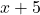which is you obtained after the polynomial long division as quotient. Let perform a polynomial long division on another polynomial.

Example #2:

Divide \hspace{3 mm} x - 1 ) \overline{x^3 + 3x^2 + 3x -4}

Solution:

\begin{aligned}
&\hspace{ 1 cm}x^2 + 4x + 7 \\
&x - 1 ) \overline{x^3 + 3x^2 + 3x - 4} \\
&\hspace{ 1 cm}x^3 - x^2  \\
&\hspace{ 1 cm}\overline{4x^2 + 3x } \\
&\hspace{ 1 cm}4x^2 - 4x\\
&\hspace{ 2 cm}\overline{7x - 4} \\
&\hspace{ 2 cm}7x - 7\\
&\hspace{ 2.7 cm}\overline{11}\\
\end{aligned}

This time the solution is a trinomial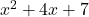. Note that the result of polynomial long division is not always zero, you may get a non-zero remainder too.

How do you write answer when the polynomial long division gives you a remainder. As in above case, you can write answers as

\frac{x^3 + 3x^2 + 3x -4}{x - 1} = x^2 + 4x + 7 + \frac{13}{x - 1}

The divisor still tries to divide the remainder so you represent it as a term.

### Division Algorithm

We can rewrite the whole dividend , divisor , quotient and remainder as an expression by itself.

x^3 + 3x^2 + 3x -4 = (x - 1)(x^2 + 4x + 7) +11

What we are doing is a check to see whether multiplying and adding the remainder back will give us the original polynomial function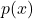Let say that the dividend is, divisor is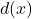, quotient is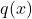and remainder is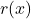.

The degree of divisoris less than or equal to, where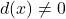. Also, there exists unique polynomialsand.

The degree of remainder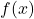is 0 or less than degree of divisor. If remainder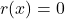, then we can say that divisordivides polynomialevenly andandare factors of the polynomial.

### Synthetic Division

Another way to divide polynomial is much faster and efficient provide that the divisor is in the form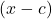where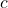is a constant.

We can take our previous example to perform the synthetic division.

Example #3

Divide using synthetic division method: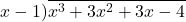Solution:

Step 1: Write the constant $c$ from the divisor and all the coefficients from the dividend.

Step 3: Write the 1st term coefficient in third column.

Step 4: Multiply 1st term coefficient with c and write the result in second column and second row and add the entries of second column. Write the result in second column, third row.

Step 5: Repeat the process from step 1 to 4.

The numbers in the last row is coefficients of quotientand remainder. Therefore, the result is

q(x) = x^2 + 4x + 7 + \frac{11}{x -1}

### Remainder Theorem

Let us remember the division algorithm, which is

f(x) = d(x) . q(x) + r

Suppose we are dividingwith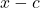, then remainder will be a constant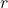, which means,

f(x) = (x - c) q(x) + r

Given the above equation, suppose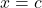, then

f(c) = (c - c)q(c) + r\\
= 0 \cdot q(c) + r\\
f(x) = r



Therefore, remainder theorem states that you can usefromand get the remainder.

Example #4

Find the remainder for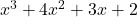divided by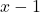Solution:

Using reminder theorem,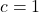and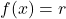.

f(1) = 1^3 + 4(1)^2 + 3(1) + 2 = 1 + 4 + 3 + 2 = 10

Therefore, remainder is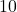.

Verify the results:

1 : 1  4  3  2
1  5  8
1  5  8  10

Using the synthetic division we find that the remainder is 10.

### Factor Theorem

The factor theorem is derived from division algorithm. Suppose thedivides the polynomial. We know that by remainder theorem,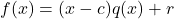which result in.

Let us replacein above equation as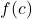. Now the division algorithm becomes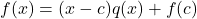.

Suppose if the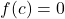then, the equation becomes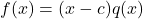which implies that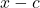is a factor of.

Let us say thatis a factor of polynomial.Then,

f(x) = (x - c) q(x) ,  if \hspace{2 mm} x = c\\
f(c) = (c - c) q(c) = 0 \cdot q(c) = 0

Ifis a factor of, then. This is known as factor theorem.

### Summary

In this article, you learned about polynomial long division, synthetic division, division algorithm and two theorem that are derived from the division algorithms – remainder theorem and factor theorem.